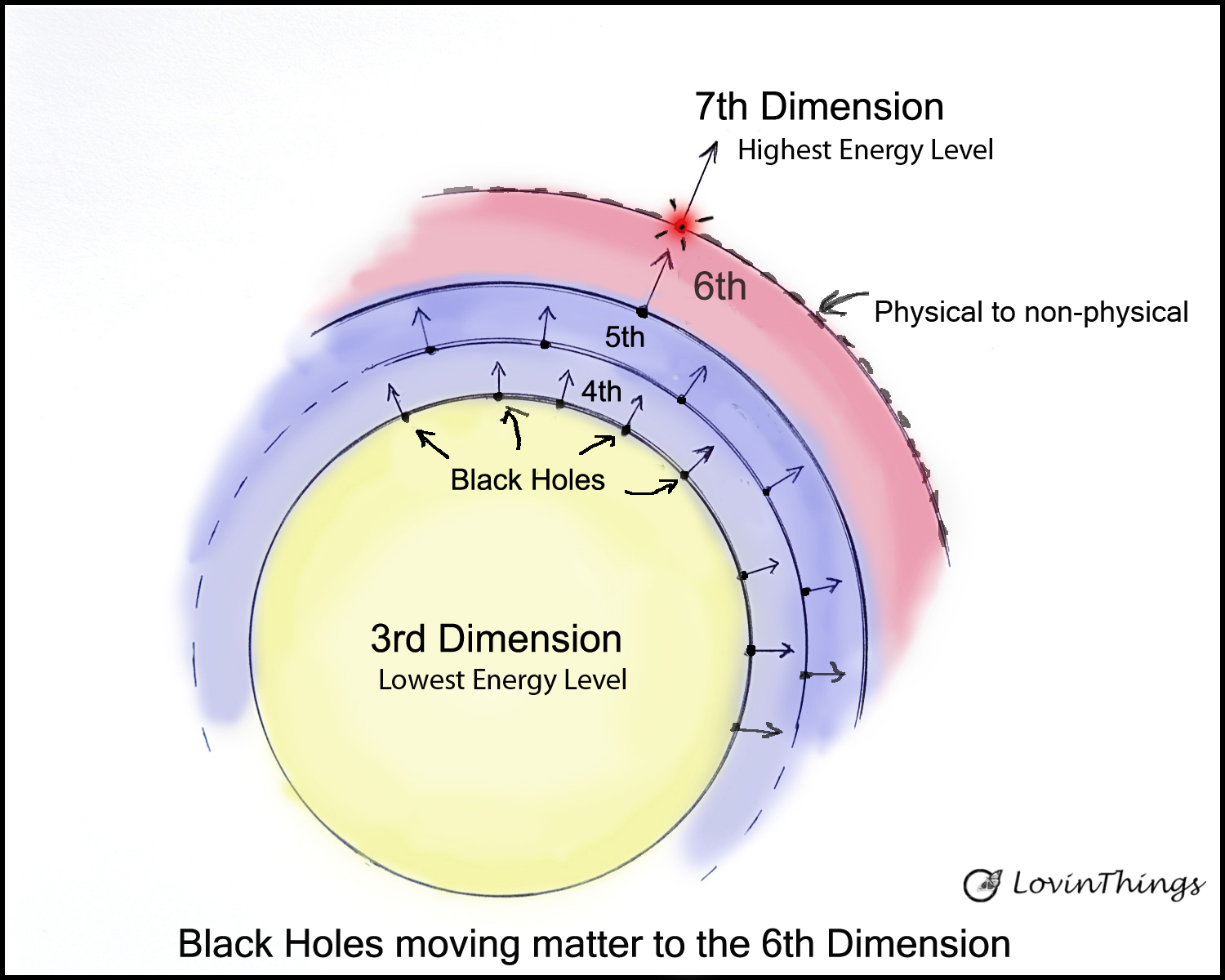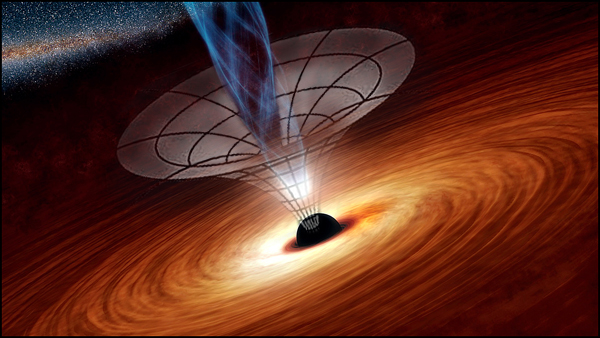# Dimensions of Space

Dimensions and dimensions of space are misunderstood. Scientists only recognize the 3-spatial dimensions which are measurements that describe things or locations. When we describe space itself it needs a different way to describe the invisible space that contains the universe.Dimensions are things that we don’t understand.

Most science fiction people think dimensions are in other realms where aliens exist.

How can we explain the dimensions of space in the universe so that even scientists can understand them?

I think we need a new way to describe the space of the universe. Using the word dimensions is not the correct description for space.

Space is a real thing that has energy so let’s call space (the container of the universe) a dimension of energy.

But what if there are multiple dimensions of energy in the universe?

How about using the words “energy levels”. The 3rd dimension would be the lowest energy level. And then the 4th energy level and so on.

Here is a diagram of the energy levels as I imagine them.The universe starts in the 3rd dimension as the lowest form of energy. Each higher dimension or energy level has higher frequency energy until it becomes nonphysical in the 7th energy level dimension.

## Is Time a Real Dimension

Time is considered as the 4th dimension in physics. However, scientists don’t know what dimensions are except in the directions and sizes of objects.

Science refuses to believe that the universe has dimensions other than the 3rd dimension.

The confusion is a result of looking for extra dimensions inside of the 3rd dimension.

Extra dimensions exist inside their own places of space. Consider that time isn’t a dimension. Time is only a measurement of motion.

Time and space are treated as a fabric of Spacetime in Einstein’s relativity. But here’s the problem, time isn’t a real thing. Is time really a dimension?

Time is a number on the clock and a number on a graph of Spacetime. Numbers aren’t real things.

Time is only a measurement of motion based on the motion of our planet.

The measure on a clock doesn’t explain what the motion is. Measuring the speed of light doesn’t explain what light is.

Measuring the force of gravity doesn’t explain what gravity is. And what about space?

How can something like Spacetime be real when it’s not made of real things?

Most scientists know that spacetime, also referred to as Minkowski Spacetime, is only a geometrical representation and not a real thing.

Spacetime is like a map of an event as shown on a drawing but the map is not the territory.

Including time as a dimension of space has made many people believe that time is real like a dimension and that time travel is possible.

Because time is only a measurement of some kind of motion we should get used to calling time what it really is.

Time is the motion of an event recorded by a clock. The number on the clock doesn’t say what the motion is or where the motion comes from.

For instance, the time of day on Earth tells us what the position of the sun is compared to the motion of the rotating Earth according to the standard Greenwich Mean Time.Where am I?

# Our Location in Space

Where are we at this moment in space?

The only reference we have is our distance to the sun during our orbit based on the angle of Earth’s rotational axis that is facing the sun.

I just quickly wrote what I was thinking at this moment of time. There might be other more relevant descriptions.

The point that I’m making is that we don’t have a GPS position because the Earth is rotating at the equator roughly 1,000 miles per hour.

And the Earth orbits the sun at the speed of nearly 30 kilometers per second, or 67,000 miles per hour.

On top of that the sun and solar system move around the Milky Way galaxy at 828,000 km per hour.

But even the Milky Way galaxy is moving in space as the universe expands.

Can you imagine the motion in the universe? It doesn’t make any sense to use Minkowski Spacetime in relation to the position of Earth.

Furthermore, the universe has many energy-levels or dimensions as well as black holes which are moving matter into other levels of energy.

My suggestion is to remove time as a dimension of space. Each of the energy-dimensions of the universe will have motion and the measure of that motion will have different times.

Each energy-dimension has the same 3-spatial dimensions and its own time frame.

Therefore, time should be on a separate graph that describes the force of motion that is being measured.

A universal Spacetime, even as a mathematical construct, has no real meaning because the motion of energy is moving so fast.

For your own sanity please stop imagining that time travel is possible.The power of gravity creates black holes and moves energy into other levels in the universe.

## Energy Levels of Space

What if the universe has many dimensions?

This would answer all the questions that scientists are asking, such as where is the dark energy, where is all the antimatter and where did the Big Bang come from?

We need another definition of dimensions! Instead of only using the 3 spatial directions to measure a dimension let’s imagine that space has energy and a frequency.

Then each dimension would be separated by a frequency difference just like radio stations are separated by different frequencies.

The radio stations are all in the same space but in different frequencies.

The entire electromagnetic spectrum is separated by frequencies so why not have dimensions that are separated by frequencies?

How can scientists prove this and listen to logic which points to the obvious truth that different dimensions of energy levels exist?

Well one way might be to apply a high enough energy to a particle and see if it disappears. Where did it go? Perhaps into another energy level.

What do you think about my idea of energy levels instead of dimensions?

Share this with friends and family who might be interested in the science of the universe.

I thank you for being here and as always be well,  https://lovinthings.com

### About the Author Erik Lovin

Erik has a BSc degree and is a retired professional photographer who is now a published Author of many books. His passion is understanding how life and the universe works. He is currently blogging about the science of the Big Bang and the science of cosmology. Erik is helping his tribe with questions about the universe. His goal is to help find a theory of everything (TOE). In order to do that, he is trying to prove light has mass and that the fabric of spacetime is a false theory. We are welcoming questions and answers that you might have about the universe.